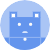search
Search
menu search toc more_vert
Guest 0reps
Thanks for the thanks!
close
Cancel
Post
account_circle
Profile
exit_to_app
Sign out
help Ask a question
search
keyboard_voice
close
Searching Tips
Search for a recipe:
"Creating a table in MySQL"
Search for an API documentation: "@append"
Search for code: "!dataframe"
Apply a tag filter: "#python"
Useful Shortcuts
/ to open search panel
Esc to close search panel
to navigate between search results
d to clear all current filters
Enter to expand content previewDoc SearchCode Search BetaSORRY NOTHING FOUND!
mic
Start speaking...Voice search is only supported in Safari and Chrome.
Shrink
Navigate to
A
A
brightness_medium
share
arrow_backShareTwitterFacebook
1
thumb_down
0
chat_bubble_outline
0
auto_stories new
settings

# Pandas DataFrame | mean method

Pandas
chevron_right
Documentation
chevron_right
DataFrame
chevron_right
Basic and Descriptive Statistics
schedule Jul 1, 2022
Last updated
local_offer PythonPandas
Tags
expand_more

Pandas `DataFrame.mean(~)` method computes the mean for each row or column of the DataFrame.

# Parameters

1. `axis`link | `int` or `string` | `optional`

Whether to compute the mean row-wise or column-wise:

Axis

Description

Mean is computed for each column.

`"index"` or `0`

Mean is computed for each row.

`"columns"` or `1`

By default, `axis=0`.

2. `skipna`link | `boolean` | `optional`

Whether or not to skip `NaN`. Skipped `NaN` would not count towards the total size, which is the divisor when computing the mean. By default, `skipna=True`.

3. `level` | `string` or `int` | `optional`

The name or the integer index of the level to consider. This is only relevant if your DataFrame is Multi-index.

4. `numeric_only`link | `None` or `boolean` | `optional`

The allowed values are as follows:

Value

Description

`True`

Only numeric rows/columns will be considered (e.g. `float`, `int`, `boolean`).

`False`

Attempt computation with all types (e.g. strings and dates), and throw an error whenever the mean cannot be computed.

`None`

Attempt computation with all types, and ignore all rows/columns whose mean cannot be computed without raising an error.

Note that means can only be computed when the `+` operator is well-defined between the types.

By default, `numeric_only=None`.

# Return Value

If the `level` parameter is specified, then a `DataFrame` will be returned. Otherwise, a `Series` will be returned.

# Examples

Consider the following DataFrame:

``` df = pd.DataFrame({"A":[2,3], "B":[4,5]})df    A  B0  2  41  3  5 ```

## Column-wise mean

To compute the mean for each column:

``` df.mean()   # or axis=0 A 2.5B 4.5dtype: float64 ```

## Row-wise mean

To compute the mean for each row, set `axis=1`:

``` df.mean(axis=1) 0 3.01 4.0dtype: float64 ```

## Specifying skipna

Consider the following DataFrame with a missing value:

``` df = pd.DataFrame({"A":[3,pd.np.nan,5]})df A0 3.01 NaN2 5.0 ```

By default `skipna=True`, which means that all missing values will be ignored when computing the mean:

``` df.mean()   # skipna=True A 4.0dtype: float64 ```

To take into account missing values:

``` df.mean(skipna=False) A NaNdtype: float64 ```

Note that if the row/column contains a missing value, then the mean for that row/column will be `NaN`.

## Specifying numeric_only

Consider the following DataFrame:

``` df = pd.DataFrame({"A":[4,5], "B":[2,True], "C":["6",False]})df    A  B     C0  4  2     "6"1  5  True  False ```

Here, both columns `B` and `C` contain mixed types, but the key difference is that summation is defined for `B`, but not for `C`. Computing the mean requires summation between the types to be well-defined.

Recall that the internal representation of a `True` boolean is `1`, so the operation `2+True` actually evaluates to `3`:

``` 2 + True 3 ```

On the other hand, `"6"+False` throws an error:

``` 6 + "False" TypeError: unsupported operand type(s) for +: 'int' and 'str' ```

### None

By default, `numeric_only=None`, which means that rows/columns with mixed types will also be considered:

``` df.mean(numeric_only=None) A 4.5B 1.5dtype: float64 ```

Here, notice how the mean was computed for column `B`, but not for `C`. By passing in `None`, rows/columns where the mean cannot be computed (due to invalid summation of types) will simply be ignored without raising an error.

### False

By setting `numeric_only=False`, rows/columns with mixed types will again be considered, but an error will be thrown when the mean cannot be computed:

``` df.mean(numeric_only=False) TypeError: can only concatenate str (not "bool") to str ```

Here, we end up with an error because column `C` contains mixed types where the `+` operation is not defined.

### True

By setting `numeric_only=True`, only numeric rows/columns will be considered:

``` df.mean(numeric_only=True) A 4.5dtype: float64 ```

Notice how columns `B` and `C` were ignored since they contain mixed types.

mail